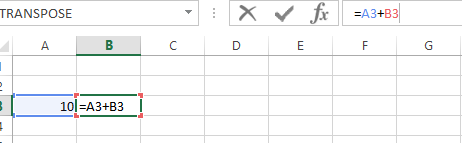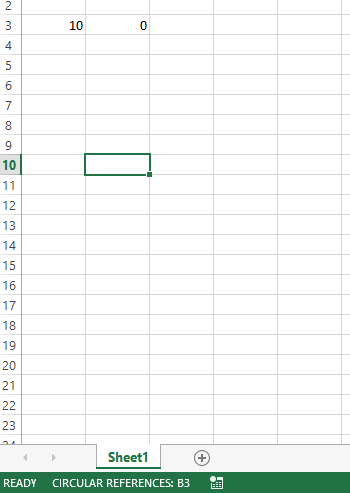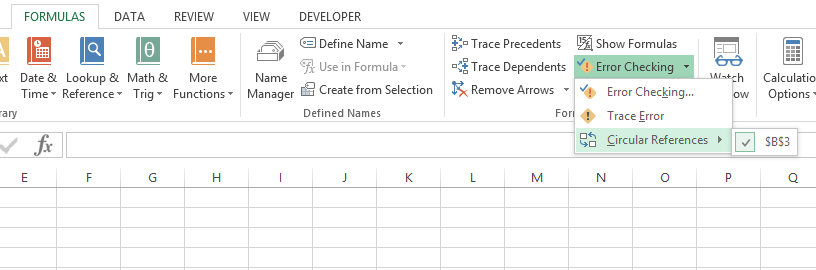### How To Find Circular References In Excel

In this Article we will learn how to find the circular references in an Excel file.

Let us understand what circular reference is. A circular reference happens and a formula directly of indirectly refers to its own cell. This causes the formula to use the result in the calculation and it runs in a loop and hence causes an error. When there are circular reference errors the Excel cannot do the other formula calculations automatically.

If you leave them in, Excel calculates each cell involved in the circular reference by using the results of the previous iteration. An iteration is a repeated recalculation until a specific numeric condition is met. By default, Excel stops calculating after 100 iterations or after all values in the circular reference change by less than 0.001 between iterations, unless you change the Excel option.

Lets create a circular reference now to look at it :

I wrote a value in cell A3 which is 10

In cell B3 I wrote a formula: = A3+B3

Now as we are already putting a formula in B3 and using the same in formula.On clicking enter/tab it gives the following error:On clicking ok it gives the wrong results = 0 and in the bottom it keeps showing warning:Now let us see how to find the circular reference:

1. Go to the Formulas tab.2.Click on error checking:In error checking click circular references:It shows the cells which has the issue and we can correct.

If you cannot figure out if the cell is the cause of the circular reference, click the next cell in the Circular References submenu, if available.

Continue to review and correct the circular reference until the status bar no longer displays the word “Circular”.

If you want to remove it see how to remove circular reference.

## Template

```Further reading: